Courses

# The Ideal Gas Equation, Dalton's Law of Partial Pressures and Mole Fraction Class 11 Notes | EduRev

## : The Ideal Gas Equation, Dalton's Law of Partial Pressures and Mole Fraction Class 11 Notes | EduRev

The document The Ideal Gas Equation, Dalton's Law of Partial Pressures and Mole Fraction Class 11 Notes | EduRev is a part of the Course Chemistry for JEE.

(v) Ideal Gas Equation

Combining all these gas laws, a simple equation can be derived at, which relates P , V , n and T for a gas

PV = nRT (for n moles of gas)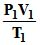=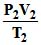(combined gas law)

P is the pressure of the gas and can be expressed in atm or Pa . Correspondingly, the volume must be expressed in litres or m3 respectively. n is the number of moles and T is the temperature in Kelvin. R is called the universal gas constant.

Numerical Values of R

(i) In litre atmosphere = 0.0821 litre atm deg-1 mole-1

(ii) In ergs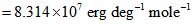(iii) In joules = 8.314 jouls deg-1 mol-1

(iv) In calories =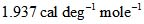Illustration 5 : An open vessel at 27°C is heated until 3/5th of the air in it has been expelled. Assuming that the volume of the vessel remains constant find

(A) the air escaped out if vessel is heated to 900K.

(B) temperature at which half of the air escapes out.

Solution : One should clearly note the fact that on heating a gas in a vessel, there are the number of moles of gas which go out, the volume of vessel remains constant.

Let initial no. of moles of gas at 300 K be `n'. On heating 3/5 moles of air escape out at temperature T.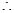Moles of air left at temperature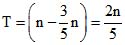(A) On heating vessel to 900 K, let n1 moles be left,

n1T1 = n2T2 ⇒ n1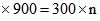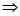moles escaped out =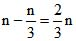moles

(B) Let n/2 moles escape out at temperature T, then

nT1 = n2 T2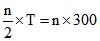⇒ T = 600 K

Illustration 6 : 5g of ethane is confined in a bulb of one litre capacity. The bulb is so weak that it will burst if the pressure exceeds 10 atm. At what temperature will the pressure of gas reach the bursting value?

Solution: PV = nRT ⇒ 10 ×1 =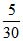× 0.082 × T

T =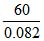= 60 × 12.18 730.81 K = 457.81ºC

(vi) Relation between Molecular Mass and Gas Densities

(A) Actual density :  For an ideal gas PV = nRT or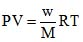, where w = mass of the gas in gms and M = Molecular wt. in gms.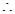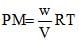or PM = RT, (where is the density of the gas =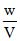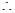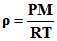(i)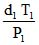=(for same gas at different temperature and pressure)

(ii)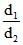=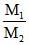(for different gases at same temperature & pressure)

(Where d = density of gas)

Illustration 7: The density of an unknown gas at 98°C and 0.974 atm is 2.5 × 10-3 g/ml. What is the mol wt. of gas?

Solution: Density =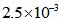g/ml = 2.5 g/LPM = ρRT

0.974 × M = 2.5 × 0.0821 × 371M = 78.18.

(B) Vapour Density: For gases another term which is often used is vapour-density. Vapour density of a gas is defined as the ratio of the mass of the gas occupying a certain volume at a certain temperature and pressure to the mass of hydrogen occupying the same volume at the same temperature and pressure i.e. W (gas) =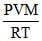.

and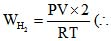mol. wt. of hydrogen is 2)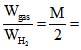(Vapour density of gas)

Vapour density of a gas is same at any temperature, pressure and volume.

Illustration 8: When 3.2 g of sulphur is vapourised at 450°C and 723 mm pressure, the vapours occupy a volume of 780 ml. What is the molecular formula of sulphur vapours under these conditions? Calculate the vapour density also.

Solutlon: PV = nRT ⇒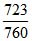×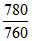=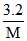× 0.082 × 723

M = 255.9

no. of c atoms of sulpher in one molecule = = 8

Molecular formula of sulphur = S8

V. D. =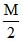=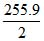127.99

(vii) Dalton's Law of Partial Pressures:

The total pressure of a mixture of non-reacting gases is equal to the sum of their partial pressures.

By Dalton's Law PT = P1 P ..............

By the partial pressure of a gas in a mixture is meant, the pressure that the gas will exert if it occupies alone the total volume of the mixture at the same temperature.

Derivation: n = n1 n2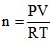⇒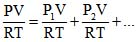⇒ P = P1 P2

Assumption: Volume of all the gases is same as they are kept in same container.

Relationship between partial pressure and number of moles

Important formula

(i)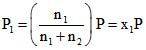where x1 = mole fraction of gas

(ii) Partial pressure of a gas in the mixture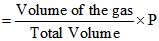Partial pressure and aqueous tension: Dalton's law is used to calculate the pressure of a dry gas when it is collected over water at atmospheric pressure.

By Dalton's law,

Pressure of dry gas = atmospheric pressure - aqueous tension

Aqueous tension depends on temperature. It increases with temperature and becomes 760 mm at 100°C.

Illustration 9: A gaseous mixture contains 55% N2, 20% O2, and 25% CO2 by mass at a total pressure of 760 mm. Calculate the partial pressure of each gas.

Solution: Total mass of the gases = 100 g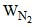= 55g,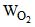= 20 g,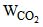= 25g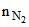= 55/28 = 1.964,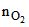= 20/32 = 0.625,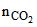= 25/44 = 0.568

Total moles = 3.157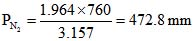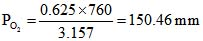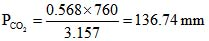Illustration 10: A mixture containing 1.6 g of O2, 1.4g of N2 and 0.4 g of He occupies a volume of 10 litre at 27°C. Calculate the total pressure of the mixture and partial pressure of each compound.

Solution: PV = nRT V = 10 litre T = 27ºC = 300K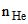=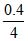= 0.1,=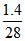= 0.05,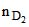=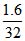= 0.05

Total no. of moles = 0.1 0.05 0.05 ⇒ 0.2

PV = nRT ⇒ P× 10 = 0.2 × 0.082 × 300 = 0.04926 ⇒ P = 0.492 atm

Partial pressure = Total pressure × molefraction

PHe = 0.492 ×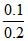= 0.246 atm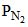= 0.492 ×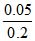= 0.123 atm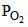= 0.492 ×= 0.123 atm

(viii) Graham's Law of Diffusion

Diffusion is the tendency of any substance to spread throughout the space available to it. Diffusion will take place in all direction and even against gravity.

The streaming of gas molecules through a small hole is called effusion.

According to Graham, the rate of diffusion (or effusion) of a gas at constant pressure and temperature is inversely proportional to the square root of its molecular mass.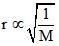, at constant P and T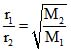, at constant P and T

Since molecular mass of gas = 2 × vapour density,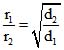, at constant P and T

The rate of diffusion (or effusion) r of two gases under different pressure can be given by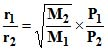at constant T only.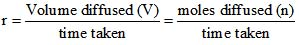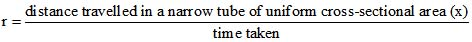Therefore, according to Graham's law of diffusion (effusion) at constant P and T.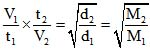dand d2 are the respective densities and V1 and V2 are volumes diffused (effused) in time t1 and t2.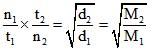where n1, n2 are moles diffused (effused) in time t1 and t2.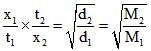where x1 and x2 are distances travelled by molecules in narrow tube in time t1 and t2.

r =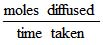=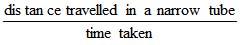=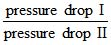Note:  It should be noted that the rate of diffusion or effusion actually depends on pressure difference of the gas and not simply on its pressure. Moreover the pressure difference is to be measured for this gas only i.e. if a container holds [He] at a pressure of 0.1 atm and if a small pin-hole is made in the container and if the container is placed in a room, then the rate of effusion of He gas from the container to outside depends only on its pressure difference, which is 0.1-0 (as their is no He in the atmosphere). This implies that the diffusion of a gas is not dependent on the diffusion of any other gas.

Whenever we consider the diffusion of gas under experimental conditions, we always assume that the gas diffuses in vacuum and during the time period for which the diffusion is studied the rate of diffusion (or the composition of diffusing or effusing mixture of gases) remains constant.

Illustration 11: Pure O2 diffuses through an aperture in 224 seconds, whereas mixture of O2 and another gas containing 80% O2 diffuses from the same in 234 sec under similar condition of pressure and temperature. What is molecular wt. of gas?

Solution: The gaseous mixture contains 80% O2 and 20% gas.

∴ Average molecular weight of mixture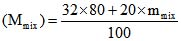… (i)

Now for diffusion of gaseous mixture and pure O2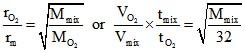or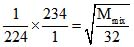… (ii)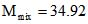By (i) and (ii) mol weight of gas (m) =46.6.

Illustration 12: Calculate the relative rates of diffusion of 235UF6 and 238UF6 in the gaseous state (Atomic mass of F = 19).

Solution: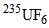and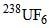(at F = 19)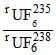=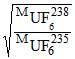=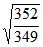= 1.0042.

Offer running on EduRev: Apply code STAYHOME200 to get INR 200 off on our premium plan EduRev Infinity!

## Chemistry for JEE

198 videos|447 docs|370 tests